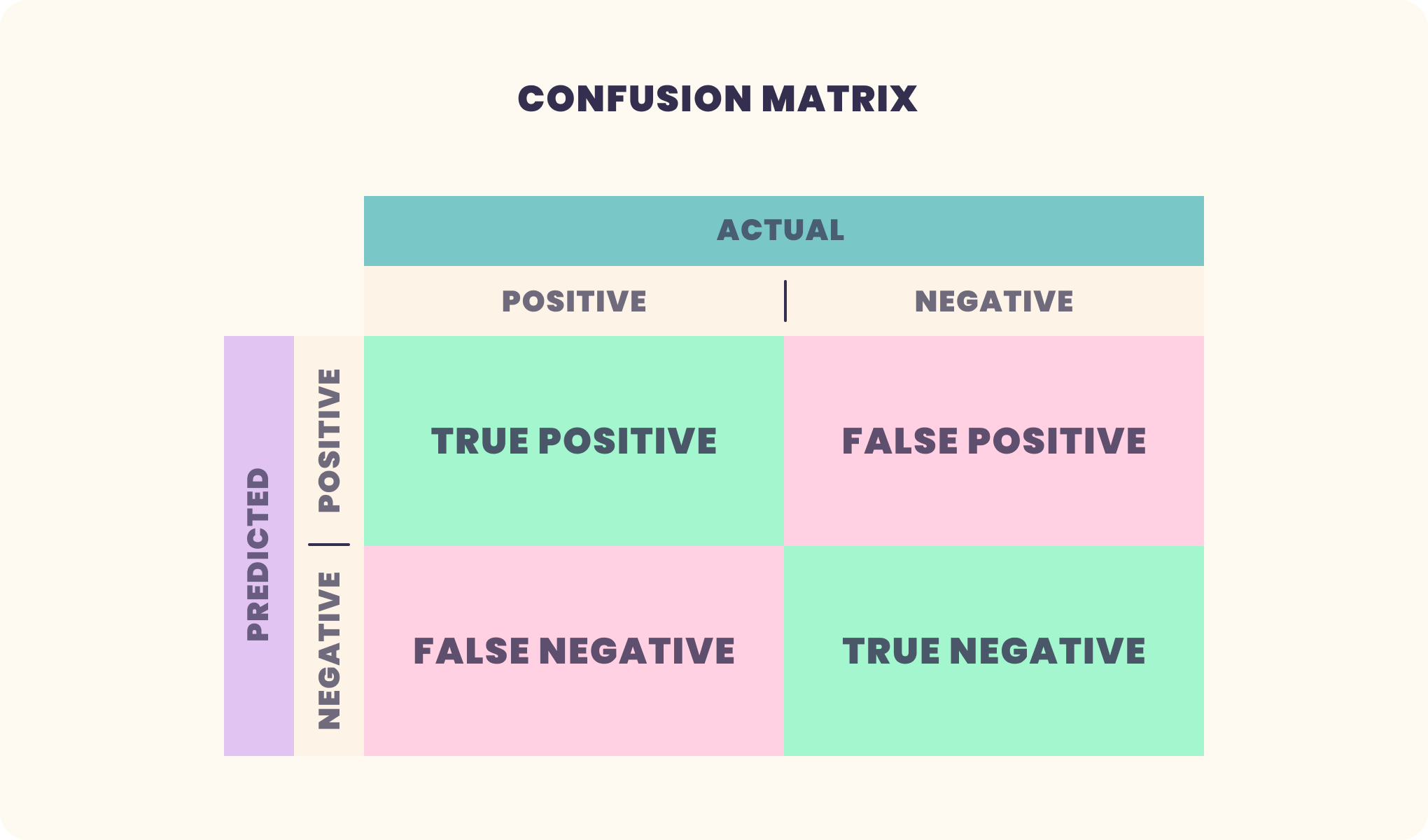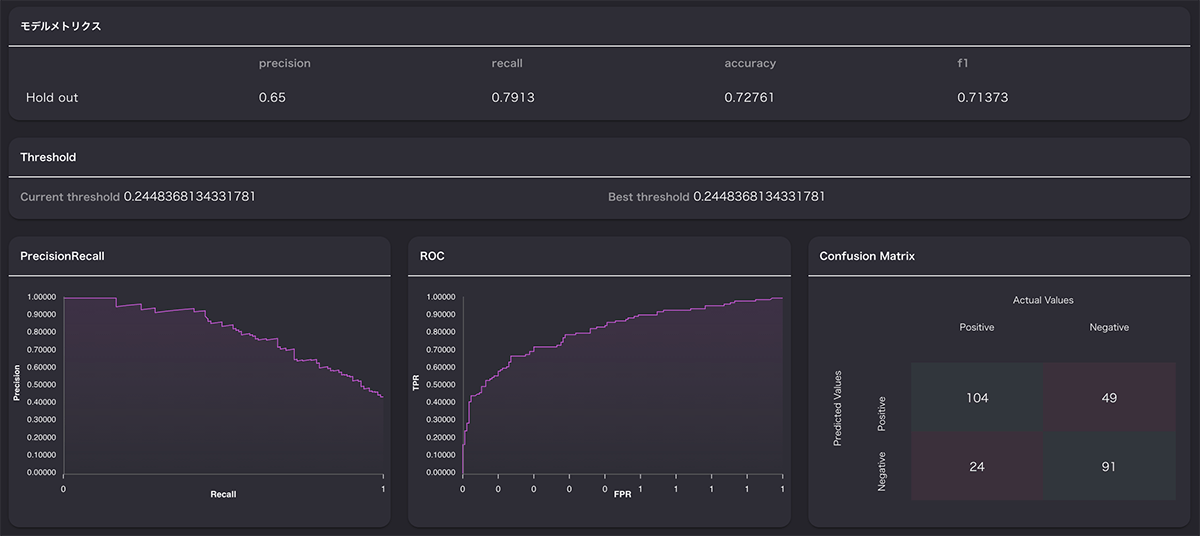Machine Learning Knowledge Center

# Confusion Matrix

## Overview

Confusion Matrix is a table of correct and incorrect predictions made by a trained model in a classification problem, as shown in the figure below.
This table is used to evaluate the performance of the created model.In this figure, the vertical axis shows the prediction results and the horizontal axis shows the actual values. Some figures show the opposite, but what they show is the same.

## Explanation of terms

Terms appearing in the figures

• True Positive [ TP ].
The number of times that data that is actually true was correctly predicted to be true.
• False Positive [ FP ].
The number of cases in which data that is actually true was predicted to be false.
• False Negative [FN].
The number of times we predicted data to be true when it was actually false
• True Negative [TN].
The number of times we correctly predicted false for data that is actually false

From this table, we can calculate the following information

• Correct rate: Accuracy
The percentage of correct predictions for all predictions.
Accuracy = ( TP + TN ) / ( TP + FP + TN + FN )
• Recall rate: Recall
Recall = TP / (TP + FN)
• Performance rate: Precision
Precision = TP / (TP + FP)
• Specificity = TN / ( FP + FN )
Specificity = TN / ( FP + TN )

## Display in VARISTA

VARISTA automatically displays the confusion matrix when the training is completed.
VARISTA can also automatically calculate and display other parameters such as Accuracy and Recall.VARISTAは機械学習モデルの開発、管理をノーコードで効率的に行うことができる新しいプラットフォームです。
データをお持ちでしたらすぐに始められますので、是非ともお試しください。

or

Stay in Touch